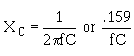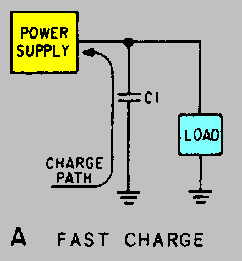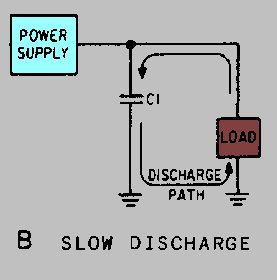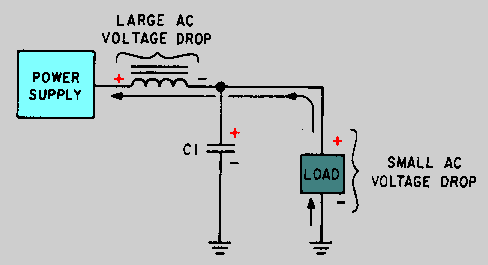filter circuits placed between the output of the rectifier and the load. There are four basic types of filter circuits: ">Custom SearchFILTERS While the output of a rectifier is a pulsating dc, most electronic circuits require a substantially pure dc for proper operation. This type of output is provided by single or multisection filter circuits placed between the output of the rectifier and the load. There are four basic types of filter circuits: Simple capacitor filter LC choke-input filter LC capacitor-input filter(pi-type) RC capacitor-input filter(pi-type) The function of each of these filters will be covered in detail in this chapter. Filtering is accomplished by the use of capacitors, inductors, and/or resistors in various combinations. Inductors are used as series impedances to oppose the flow of alternating (pulsating dc) current. Capacitors are used as shunt elements to bypass the alternating components of the signal around the load (to ground). Resistors are used in place of inductors in low current applications. Let's briefly review the properties of a capacitor. First, a capacitor opposes any change in voltage. The opposition to a change in current is called capacitive reactance (XC) and is measured in ohms. The capacitive reactance is determined by the frequency (f) of the applied voltage and the capacitance (C) of the capacitor.From the formula, you can see that if frequency or capacitance is increased, the XC decreases. Since filter capacitors are placed in parallel with the load, a low XC will provide better filtering than a high XC. For this to be accomplished, a better shunting effect of the ac around the load is provided, as shown in figure 4-10. To obtain a steady dc output, the capacitor must charge almost instantaneously to the value of applied voltage. Once charged, the capacitor must retain the charge as long as possible. The capacitor must have a short charge time constant (view A). This can be accomplished by keeping the internal resistance of the power supply as small as possible (fast charge time) and the resistance of the load as large as possible (for a slow discharge time as illustrated in view B). Figure 4-10A. - Capacitor filter. FAST CHARGEFigure 4-10B. - Capacitor filter. SLOW DISCHARGEFrom your earlier studies in basic electricity, you may remember that one time constant is defined as the time it takes a capacitor to charge to 63.2 percent of the applied voltage or to discharge to 36.8 percent of its total charge. This action can be expressed by the following equation: t = RC Where: R represents the resistance of the charge or discharge path And: C represents the capacitance of the capacitor. You should also recall that a capacitor is considered fully charged after five RC time constants. Refer to figure 4-11. You can see that a steady dc output voltage is obtained when the capacitor charges rapidly and discharges as slowly as possible. Figure 4-11. - RC time constant.In filter circuits the capacitor is the common element to both the charge and the discharge paths. Therefore, to obtain the longest possible discharge time, you want the capacitor to be as large as possible. Another way to look at it is: The capacitor acts as a short circuit around the load (as far as the ac component is concerned), and sincethe larger the value of the capacitor (C), the smaller the opposition (XC) or reactance to ac. Now let's look at inductors and their application in filter circuits. Remember, AN INDUCTOR OPPOSES ANY CHANGE IN CURRENT. In case you have forgotten, a change in current through an inductor produces a changing electromagnetic field. The changing field, in turn, cuts the windings of the wire in the inductor and thereby produces a counter electromotive force (CEMF). It is the CEMF that opposes the change in circuit current. Opposition to a change in current at a given frequency is called inductive reactance (XL) and is measured in ohms. The inductive reactance (XL) of an inductor is determined by the applied frequency and the inductance of the inductor. Mathematically, XL = 2pfL If frequency or inductance is increased, the XL increases. Since inductors are placed in series with the load (as shown in figure 4-12), the larger the XL, the larger the ac voltage developed across the load. Figure 4-12. - Voltage drops in an inductive filter.Now refer to figure 4-13. When the current starts to flow through the coil, an expanding magnetic field builds up around the inductor. This magnetic field around the coil develops the CEMF that opposes the change in current. When the rectifier current decreases, as shown in figure 4-14, the magnetic field collapses and again cuts the turns (windings) of wire, thus inducing current into the coil. This additional current merges with the rectifier current and attempts to keep it at its original level. Figure 4-13. - Inductive filter (expanding field).Figure 4-14. - Inductive filter (collapsing field).Now that you have read how the components in a filter circuit react to current flow from the rectifier, the different types of filter circuits in use today will be discussed. Q.13 If you increase the value of the capacitor, will the XC increase or decrease? Why?Integrated Publishing, Inc. - A (SDVOSB) Service Disabled Veteran Owned Small Business